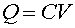Definition of Capacitance

A capacitor is an electronic component used for storing charge and energy. The usual capacitor is a pair of parallel plates separated by a small distance. When a steady voltage is applied across a capacitor, a charge +Q is stored on one plate while -Q is stored on the opposite plate. The amount of charge is determined by the capacitance C and the voltage difference V applied across the capacitor:Since the charge cannot change instantaneously, the voltage across a capacitor cannot change instantaneously either. Thus capacitors can be used to guard against sudden losses of voltage in circuits, among other uses that we will study later.

Capacitance is measured in farads. One farad (F) equals one coulomb per volt.

One of the many uses for capacitors is in computer memories. A typical computer memory chip might contain 16,777,216 capacitors; each capacitor is charged to approximately 5 volts to store the binary digit 1, or 0 volts to store the binary digit 0.

Another use of capacitors is to store energy for relatively brief times; for example, the calculator I use is powered by light energy instead of a battery, and it has a capacitor to provide power during brief intervals in which a shadow passes across its photocell.